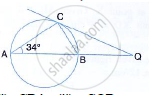Share

In the Given Figure, Ab is the Diameter. the Tangent at C Meets Ab Produced at Q. If ∠Cab = 34°, Find: (I)∠Cba (Ii) ∠Cqb - ICSE Class 10 - Mathematics

ConceptChord Properties - a Straight Line Drawn from the Center of a Circle to Bisect a Chord Which is Not a Diameter is at Right Angles to the Chord

Question

In the given figure, AB is the diameter. The tangent at C meets AB produced at Q.
If ∠CAB = 34°, Find:(i)∠CBA (ii) ∠CQB

Solution

i) AB is diameter of circle.
∴  ACB = 90°
In ΔABC,
∠A + B + ∠C = 180°
⇒  34° +  ∠CBA + 90° =  180°
⇒  ∠CBA =  56°
ii) QC is tangent to the circle
∴ ∠CAB  = ∠QCB
Angle between tangent and chord = angle in alternate segment
∴  ∠QCB =  34°
ABQ is a straight line

⇒  ∠ABC + ∠CBQ = 180°
⇒ 56° +  ∠CBQ = 180°

⇒ CBQ = 124°
Now,
∠CQB  = 180° -  ∠QCB  = CBQ
⇒  ∠CQB  = 180° - 34°- 124°
⇒  ∠CQB   =  22°

Is there an error in this question or solution?

Video TutorialsVIEW ALL 

Solution In the Given Figure, Ab is the Diameter. the Tangent at C Meets Ab Produced at Q. If ∠Cab = 34°, Find: (I)∠Cba (Ii) ∠Cqb Concept: Chord Properties - a Straight Line Drawn from the Center of a Circle to Bisect a Chord Which is Not a Diameter is at Right Angles to the Chord.
S